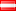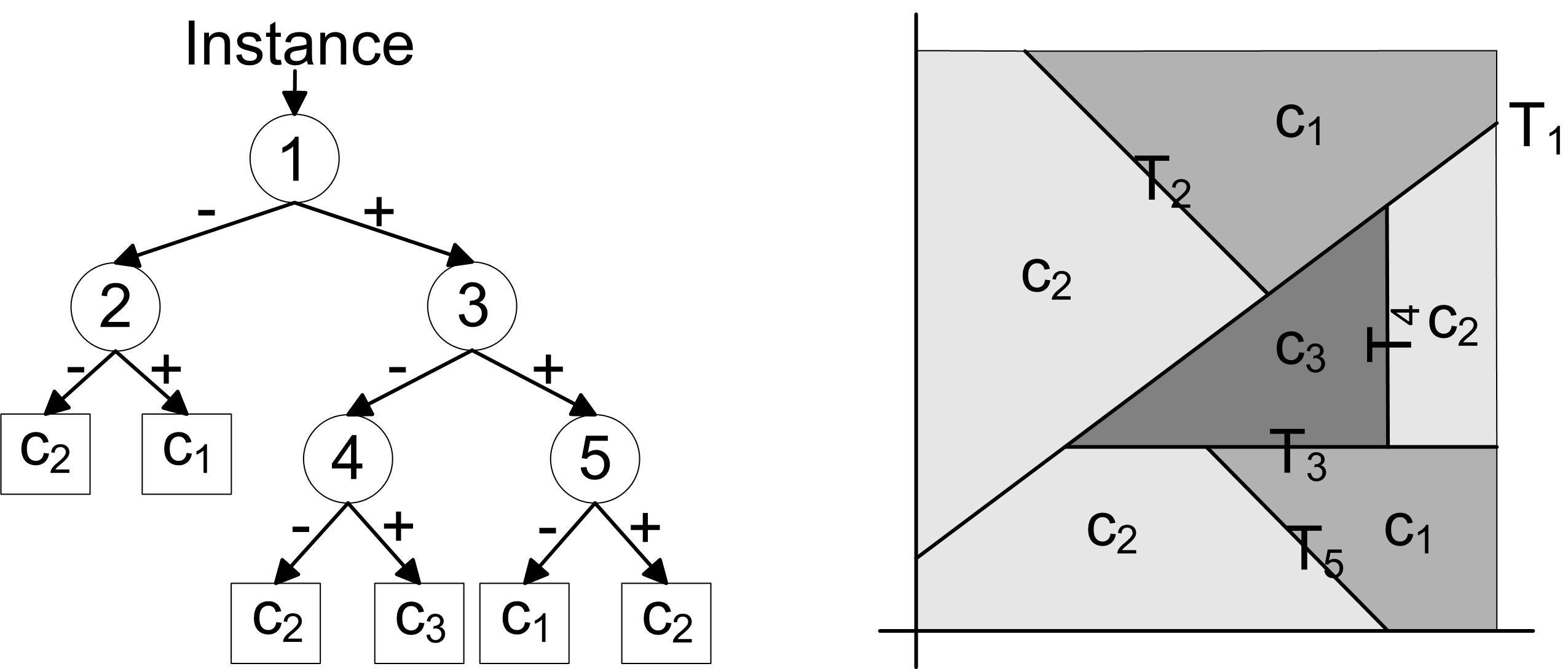# Decision Trees CoresDecision Trees (DTs) are rooted tree structures, with leaves representing classifications and nodes representing tests of features that lead to those classifications.

Although not as popular as ANN classifiers, DTs have several important advantages when compared with ANNs.

Although DTs can be less accurate than ANNs, DT learning algorithms are much faster, have smaller number of free parameters to be fine-tuned and require little data preparation, in comparison with ANN learning algorithms. In addition to that, apart from being simple to understand and interpret, DTs could be directly converted into the set of if-then rules, which is not the case with ANNs. DTs are also robust and scale well with large data sets.

In DT learning, target function is represented by a decision tree of finite depth. Every node of the tree specifies a test involving one or more attributes of the function to be learned, and every branch descending from a node matches one of the possible outcomes of the test in the considered node. To classify an instance we perform a sequence of tests associated to the sequence of nodes, starting with the root node and terminating with a leaf node. If we allow numerical attributes only, the resulting DT will be binary. The majority of algorithms allow only numerical attributes. This concept is illustrated in the Figure below.Basic structure of a decision tree with the possible classification regions in the case of two attribute classification problem.

In the above figure a structure of a typical DT is shown. In this example a classification problem with two attributes (that correspond to the x and y axes in the graph on the right) and three possible classes (c1, c2, c3) is assumed. Two dimensional attribute space is divided into classification regions by the DT using five linear tests (that correspond to the linear segments marked as T1, …, T5 on the graph). Each of these test is located in one of the DT nodes (marked with numbers 1, …, 5 in the DT) shown on the figure. Although in this example linear test have been used, DTs, in general, can also use any form of nonlinear tests to divide the classification space.

DTs are typically implemented in software. But in applications that require rapid classification or DT creation, hardware implementation is the only solution.

So-Logic is one of the first companies to offer IP cores that enable implementation of decision trees directly in hardware. Our portfolio of DT IP cores includes:

 Updated at: to the top